# Introduction

Line Labelling is applicable when two-dimensional lines have been extracted from a two-dimensional image.
Initially, we shall restrict ourselves to polyhedral objects in our scene, i.e. have flat faces bounded by straight edges.
Under this assumption it becomes possible to interpret the edges
• to determine which edges bound which faces, and
• to deduce certain relationships between faces.

Consider the line drawing shown in Fig. 38.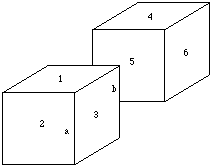Fig. 38 Line drawing of two cubes
It can be seen that
• the edges bound the numbered faces, and that faces 1, 2 and 3 belong to one cube,
• while faces 4, 5 and 6 belong to another (assuming that the two cubes are not joined by a thin sliver that cannot be seen).

An important observation is that
• Every three-dimensional edge is associated with exactly two faces -- one on either side of it,
• A few rather ill-conditioned objects called non-manifold objects are exceptions -- we shall not consider these further here.

Sometimes both of these faces can be seen from a given viewpoint (as in the case of line a in Fig. 38).
If only one of the faces is visible -- the edge is called an occluding edge. On the other side of an occluding edge, the background or a non-touching face is visible.
The basis of the line labelling method is to attempt to classify each edge in the image as being one of:
• a concave edge,
• a convex edge,
• an occluding edge.

Edges of each type are shown in Fig. 39.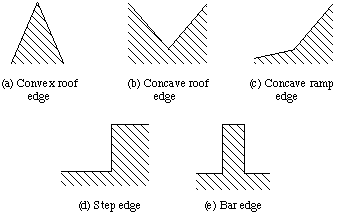Fig. 39 Types of edge in a line drawing
Where edges meet only certain possible interpretations of the meeting lines are mutually consistent.
This reduces the number of possibilities for the classification of each edge.
If a line corresponds to an occluding edge:
• It is marked with an arrow. The orientation of the arrow gives the side of the edge on which the visible face lies, using the convention that the visible face lies on the right hand side of the edge, looking along the direction of the arrow.
• Lines corresponding to convex edges are marked with a `+' symbol.
• Lines corresponding to concave edges are marked with a `-' symbol.
• Each edge in the image thus has one of four possible labellings.

# Boundary Representation

• Models are a more explicit representation than CSG.
• The object is represented by a complicated data structure giving information about each of the object's faces, edges and vertices and how they are joined together.
• Appears to be a more natural representation for Vision since surface information is readily available.
• The description of the object can be into two parts:

Topology
-- records the connectivity of the faces, edges and vertices by means of pointers in the data structure.
Geometry
-- describes the exact shape and position of each of the edges, faces and vertices.
• The geometry of a vertex is just its position in space as given by its (x,y,z) coordinates.
• Edges may be straight lines, circular arcs, etc..
• A face is represented by some description of its surface (algebraic or parametric forms used).

# Contours

For binary images, the difference between a dilated image and its original gives the external contour of the image. The structure element dictates the topology of the contour. The most widely used structure elements are the 3x3 cross to generate 4-connected contour, and a 3x3 box to generate 8-connected contour.
There are other contours that can be created, i.e., the difference between the original and the eroded image, and the difference between the dilated and eroded image.
Below is the original image and its contour taken as the subtraction between the original and the eroded image by a cross.
Original image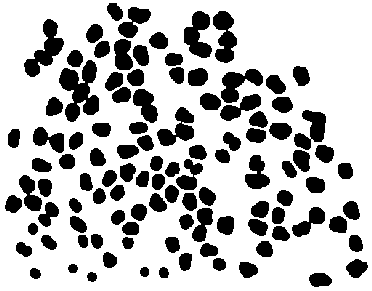Original - Eroded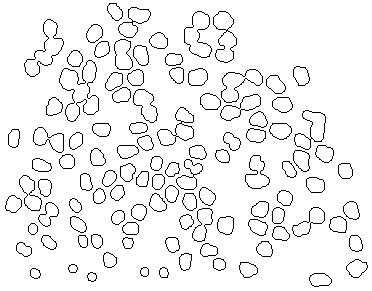We can label the contour and take its image histogram to extract the perimeter related to each connected contour.
Labeled contour image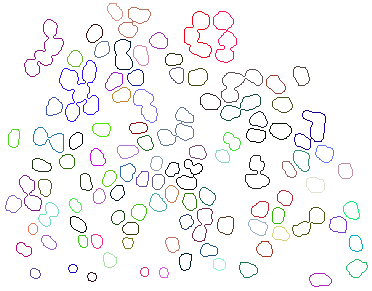Perimeter for each connected contour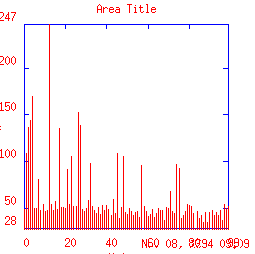# Junction Types

It is now possible to say that all line junctions will belong to one of four types, as shown in Fig. 40.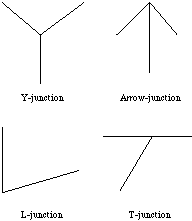Fig. 40 Types of junction in a line drawing
• At an L-junction only two edges meet,
• Three edges meet at the other junction types.
• The distinction between an Arrow-junction and a Y-junction is whether the three edges subtend a total angle of less or more than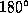.
• The dividing case between an Arrow-junction and a Y-junction is specifically identified as a T-junction.

Consideration of what such junctions can represent in a view of a polyhedral model leads to the conclusion that only certain labellings of the edges at a junction can arise in images of a real scene. These are illustrated in Fig. 41.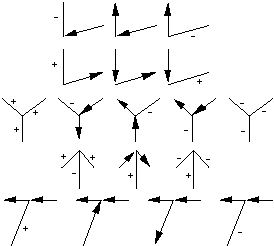Fig. 41 Permissible labellings at a junction
There are far fewer of these than the total number of all possible labellings. Some of the permissible labellings are shown occurring in a scene in Fig. 42.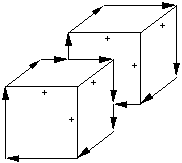Fig. 42 Labelled lines in an image
One immediate deduction that can be made is that T-junctions only occur where one object occludes another, or perhaps an object occludes another part of itself.

# Wireframe Models

• Store edges and vertices in a list.
• Polyhedral representation.
• Edge based matching.
• Problems with ambiguous (Fig. 46) and impossible (Fig. 47) representations.
• Problems due to no solid matter of the object represented.
• Solid Models are better.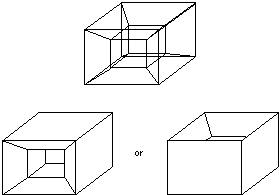Fig. 46 Ambiguous Wireframe Model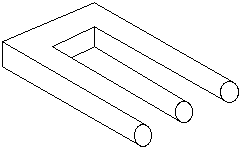# Template Matching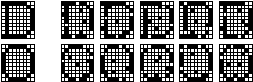Template matching is a natural approach to pattern classification. For example, consider the noisy "D"'s and "O"'s shown above. The noise-free versions shown at the left can be used as templates. To classify one of the noisy examples, simply compare it to the two templates. This can be done in a couple of equivalent ways:
1. Count the number of agreements (black matching black and white matching white). Pick the class that has the maximum number of agreements. This is a maximum correlation approach.

2. Count the number of disagreements (black where white should be or white where black should be). Pick the class with the minimum number of disagreements. This is a minimum error approach.
Template matching works well when the variations within a class are due to "additive noise." Clearly, it works for this example because there are no other distortions of the characters -- translation, rotation, shearing, warping, expansion, contraction or occlusion. It will not work on all problems, but when it is appropriate it is very effective. It can also be generalized in useful ways.

# Set-Theoretic Modelling

• Also widely known as computational solid geometry (CSG).
• Model is composed of primitive shapes, such as rectangular boxes, spheres, cylinders and cones,
• Assembled with set operators
• Represent model as a tree structure (Fig. 48).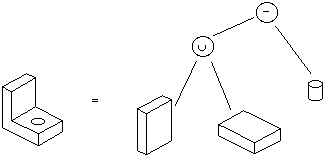Fig. 48 A set-theoretic model
• Set operators used similar to those of Boolean logic.
• Operators used are:
• union -- Boolean OR,
• intersection -- Boolean AND,
• difference -- Boolean NOT,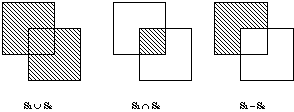# Footnote

Another natural approach to classification is the decision tree or decision table. For example, one might use the following decision tree to distinguish the upper-case letters "A", "B", "C" and "D":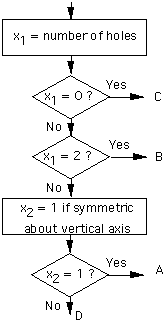This method works best when there is no uncertainty in the feature values. It can be extended by employing "fuzzy features". For example, if the figure contains one large hole and one very small hole, it might be possible to give a score or fuzzy number or probability value between 0 and 1 as an answer to the question "Is the number of holes equal to zero?". Then one might choose some threshold, so that the answer is "Yes" if the score gets above the threshold. Alternatively, if the score falls in some "gray area", one might follow more than one path through the tree and develop scores for the various terminal nodes.

Decision trees are appealing for their simplicity and efficiency. However, they tend to become unmanageable for procesing sensory input data. When there is significant uncertainty, it is usually preferable to use a procedure designed for uncertainty than to "patch up" a decision tree by embellishing it with extra, ad hoc mechanisms.